# Introduction to Spatial Data and ggplot2

http://spatial.ly/2013/12/introduction-spatial-data-ggplot2/

See [social-statistics.org/R Tutorials]: (http://www.social-statistics.org/?page_id=1085)

by James Cheshire, UCL Centre for Advanced Spatial Analysis and Robin Lovelace, University of Sheffield

For those starting out with spatial data in R, Robin Lovelace and I have prepared this tutorial (funded as part of the University of Leeds and UCL Talisman project). Here we introduce a range of analysis skills before demonstrating how you can deploy the powerful graphics capabilities of ggplot2 to visualise your results. There is also some “bonus” material at the end to show how you can use ggplot2 for descriptive statistics and so on. The tutorial covers:

• Introduction to ggplot2
• Map projections
• Clipping and joining spatial data
• Aggregating spatial data
• ggplot2 for descriptive statistics

This is a work in progress so we may add improvements as time goes on. We also have a few more tutorials in the pipeline that will be posted here in due course.

### Introduction

This tutorial is an “Introduction to Spatial Data and ggplot2” and assumes no prior knowledge of spatial data analysis in R. We do recommend users are acquainted with the R command line before beginning the practicals though, perhaps via an 'Introduction to R' type tutorial, such as “A (very) short introduction to R” (Torfs and Brauer, 2012) or the more geographically inclined “Short introduction to R” (Harris, 2012).

Building on such background material, the following set of exercises is concerned with specific functions for spatial data and also the use of a package called ggplot2 for data visualisation. An up-to-date version of this document is maintained at https://github.com/Robinlovelace/Creating-maps-in-R. Suggested improvements welcome.

Typographic conventions

To ensure reproducibility and allow automatic syntax highlighting, this document has been written in `RMarkdown`. Be aware of the following typographic conventions: R code (e.g. `plot(x, y)`) is written in a monospace font while prose is not. Blocks of code such as,

`c(1:3, 5)^2`

`##  1 4 9 25`

are compiled in-line: the `##` indicates this is output from R. Some of the output from the code below is quite long; we only show the output that is useful. A single hash (`#`) is a comment for humans to read that R will ignore.

All images in this document are small and low-quality to save space; they should display better on your computer screen and can be saved at any resolution. The code presented here is not the only way to do things: we encourage you to play with it and try things out to gain a deeper understanding of R. Don't worry, you cannot 'break' anything using R and all the input data can be re-loaded if things do go wrong.

### Prerequisites and packages

For this tutorial you need to install R, the latest version of which can be downloaded from http://cran.r-project.org/. A number of R editors such as RStudio can be used to make R more user friendly, but these are not needed to complete the tutorial.

R has a huge and growing number of spatial data packages. These can be installed in one go with the ctv package and the command `install.views("Spatial")`. We do NOT recommend running this command for this tutorial: partly because downloading and compiling all spatial packages takes a long time (hundreds of megabytes) and also because we will add new packages when they are needed to see what each does. We do recommend taking a quick browse at the range of spatial packages on offer though: http://cran.r-project.org/web/views/Spatial.html.

The packages we will be using are `ggplot2`, `rgdal`, `rgeos`, `maptools` and `ggmap`. To test whether ggplot2 is installed, for example, enter library(ggpot2). If you get an error message, it needs to be installed: `install.packages("ggplot2")`.

``````# install.packages('maptools') # uncomment to install

# install.packages('rgdal') # uncomment to install

# install.packages('rgeos') # uncomment to install

# install.packages('ggmap') # uncomment to install
``````

All of the data used for the tutorial can be downloaded from here:

Save this to a new folder, then in R specify the path of that folder as you working directory. Use the `setwd` command to do this. If your username is “`username`” and you saved the files into a folder called “`rmapping`” on your Desktop, for example, you would type the following:

``````setwd("C:/Users/username/Desktop/rmapping/R")
``````

If you are working in RStudio, you can create a project that will automatically set your working directory.

One of the most important steps in handling spatial data with R is the ability to read in shapefiles. There are a number of ways to do this. The most simple is `readShapePoly()` in the maptools package:

``````# library(maptools) # load the package
``````

This method works OK, but it is no longer considered best practice since it doesn’t load in the spatial referencing information etc associated with the shapefile. A more powerful way to read in geographical data is to use the `rgdal` function `readOGR`, which automatically extracts this information. This is R’s interface to the “Geospatial Abstraction Library (GDAL)” which is used by other open source GIS packages such as QGIS and enables R to handle a broader range of spatial data formats.

``````library(rgdal)
``````
``````## Loading required package: sp
## rgdal: version: 0.8-14, (SVN revision 496)
## Geospatial Data Abstraction Library extensions to R successfully loaded
## Loaded GDAL runtime: GDAL 1.10.1, released 2013/08/26
## Path to GDAL shared files: C:/Users/Mark/Documents/R/win-library/3.0/rgdal/gdal
## GDAL does not use iconv for recoding strings.
## Loaded PROJ.4 runtime: Rel. 4.8.0, 6 March 2012, [PJ_VERSION: 480]
## Path to PROJ.4 shared files: C:/Users/Mark/Documents/R/win-library/3.0/rgdal/proj
``````
``````sport <- readOGR(dsn = ".", "london_sport")
``````
``````## OGR data source with driver: ESRI Shapefile
## Source: ".", layer: "london_sport"
## with 33 features and 4 fields
## Feature type: wkbPolygon with 2 dimensions
``````

In the code above dsn is an argument of the function readOGR. R functions have a default order of functions, so `dsn =` does not actually need to be typed: `readOGR(".", "london_sport")` works the same, but it is good to remember the meaning of each argument when beginning to use R, so we sometimes include argument names when it is relevant. Here, dsn stands for “data source name” which is the folder containing the spatial data – this was pre-specified when you set your working directory. The next argument is a character string, text identifying the file required. There is no need to add a file extension.

The file contains the borough population and the percentage of the population engaging in sporting activities and was taken from the active people survey. The boundary data is from the Ordnance Survey.

All shapefiles have an attribute table. This is loaded with `readOGR` and can be treated in a similar way to an R data frame.

R hides the geometry of spatial data unless you print the object (using the `print()`). Let's take a look at the headings of sport, using the following command: `names(sport)` The data contained in spatial data are kept in a 'slot' that can be accessed using the `@` symbol: `sport@data`. This is useful if you do not wish to work with the spatial components of the data at all times.

Type `summary(sport)` to get some additional information about the data object. Spatial objects in R contain a variety of additional information:

``````summary(sport)
``````
``````## Object of class SpatialPolygonsDataFrame
## Coordinates:
##      min    max
## x 503571 561941
## y 155851 200932
## Is projected: TRUE
## proj4string :
## [+proj=tmerc +lat_0=49 +lon_0=-2 +k=0.9996012717 +x_0=400000
## +y_0=-100000 +ellps=airy +units=m +no_defs]
## Data attributes:
##    ons_label                    name      Partic_Per      Pop_2001
##  00AA   : 1   Barking and Dagenham: 1   Min.   : 9.1   Min.   :  7181
##  00AB   : 1   Barnet              : 1   1st Qu.:17.6   1st Qu.:181284
##  00AC   : 1   Bexley              : 1   Median :19.4   Median :216505
##  00AD   : 1   Brent               : 1   Mean   :20.1   Mean   :217335
##  00AE   : 1   Bromley             : 1   3rd Qu.:21.7   3rd Qu.:248917
##  00AF   : 1   Camden              : 1   Max.   :28.4   Max.   :330584
##  (Other):27   (Other)             :27
``````

In the above code `proj4string` represents the coordinate reference system used in the data. In this file it has been incorrectly specified so we can change it with the following:

``````proj4string(sport) <- CRS("+init=epsg:27700")
``````
``````## Warning: A new CRS was assigned to an object with an existing CRS:
## +proj=tmerc +lat_0=49 +lon_0=-2 +k=0.9996012717 +x_0=400000 +y_0=-100000 +ellps=airy +units=m +no_defs
## without reprojecting.
## For reprojection, use function spTransform in package rgdal
``````

You will see you get a warning. This is simply saying that you are changing the coordinate reference system, not reprojecting the data. `Epsg:27700` is the code for British National Grid. If we wanted to reproject the data into something like `WGS84` for latitude and longitude we would use the following code:

``````sport.wgs84 <- spTransform(sport, CRS("+init=epsg:4326"))
``````

The different `epsg` codes are a bit of hassle to remember but you can find them all at spatialreference.org.

### ggplot2

This next section of the practical introduces a slightly different method of creating plots in R using the ggplot2 package. The package is an implementation of the Grammar of Graphics (Wilkinson 2005) - a general scheme for data visualization that breaks up graphs into semantic components such as scales and layers. ggplot2 can serve as a replacement for the base graphics in R (the functions you have been plotting with today) and contains a number of default options that match good visualisation practice.

The maps we produce will not be that meaningful - the focus here is on sound visualisation with R and not sound analysis (obviously the value of the former diminished in the absence of the latter!) Whilst the instructions are step by step you are encouraged to deviate from them (trying different colours for example) to get a better understanding of what we are doing.

ggplot2 is one of the best documented packages in R. The full documentation for it can be found online and it is recommended you test out the examples on your own machines and play with them: http://docs.ggplot2.org/current/.

Good examples of graphs can also be found on the website cookbook-r.com.

``````library(ggplot2)
``````

It is worth noting that the basic `plot()` function requires no data preparation but additional effort in colour selection/adding the map key etc. `qplot()` and `ggplot()` (from the ggplot2 package) require some additional steps to format the spatial data but select colours and add keys etc automatically. More on this later.

As a first attempt with ggplot2 we can create a scatter plot with the attribute data in the sport object created above. Type:

``````p <- ggplot(sport@data, aes(Partic_Per, Pop_2001))
``````

What you have just done is set up a ggplot object where you say where you want the input data to come from. `sport@data` is actually a data frame contained within the wider spatial object sport (the `@` enables you to access the attribute table of the sport shapefile). The characters inside the aes argument refer to the parts of that data frame you wish to use (the variables `Partic_Per` and `Pop_2001`). This has to happen within the brackets of aes(), which means, roughly speaking 'aesthetics that vary'.

If you just type `p` and hit enter you get the error `No layers in plot`. This is because you have not told ggplot what you want to do with the data. We do this by adding so-called “geoms”, in this case `geom_point()`.

``````p + geom_point()
``````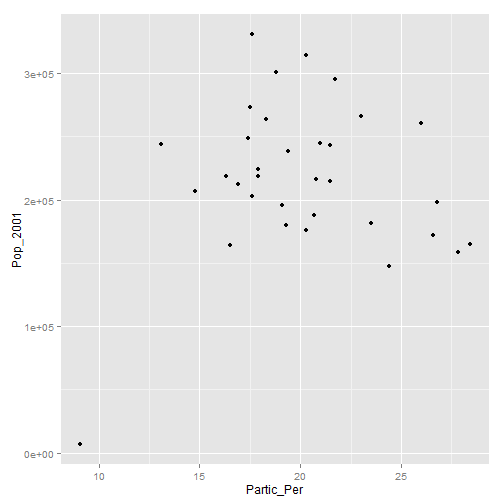Within the brackets you can alter the nature of the points. Try something like `p + geom_point(colour = "red", size=2)` and experiment.

If you want to scale the points by borough population and colour them by sports participation this is also fairly easy by adding another `aes()` argument.

``````p + geom_point(aes(colour = Partic_Per, size = Pop_2001))
``````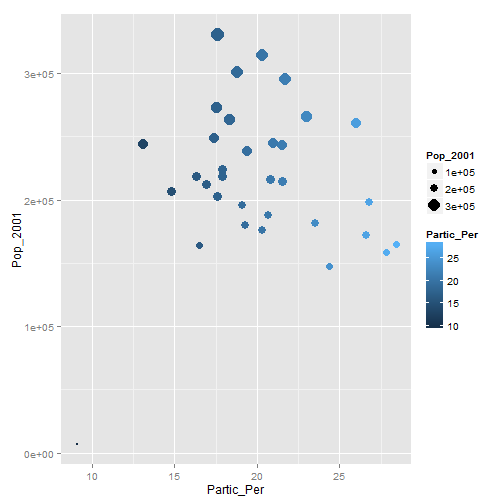The real power of ggplot2 lies in its ability to add layers to a plot. In this case we can add text to the plot.

``````p + geom_point(aes(colour = Partic_Per, size = Pop_2001)) + geom_text(size = 2,
aes(label = name))
``````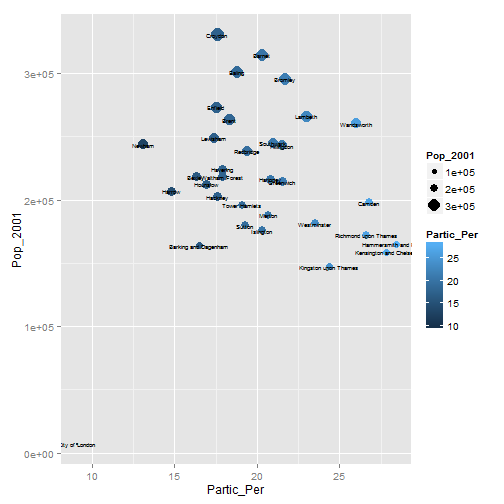This idea of layers (or geoms) is quite different from the standard plot functions in R, but you will find that each of the functions does a lot of clever stuff to make plotting much easier (see the documentation for a full list).

The following steps will create a map to show the percentage of the population in each London Borough who regularly participate in sports activities.

To get the shapefiles into a format that can be plotted we have to use the `fortify()` function. Spatial objects in R have a number of slots containing the various items of data (polygon geometry, projection, attribute information) associated with a shapefile. Slots can be thought of as shelves within the data object that contain the different attributes. The “polygons” slot contains the geometry of the polygons in the form of the XY coordinates used to draw the polygon outline. The generic plot function can work out what to do with these, ggplot2 cannot. We therefore need to extract them as a data frame. The fortify function was written specifically for this purpose. For this to work, either gpclib or rgeos packages must be installed.

``````# library(gpclib); gpclibPermit() # uncomment if rgeos not installed
sport.f <- fortify(sport, region = "ons_label")  # maptools package required for this functionality
``````
``````## Loading required package: rgeos
## rgeos version: 0.3-2, (SVN revision 413M)
##  GEOS runtime version: 3.4.2-CAPI-1.8.2 r3921
##  Polygon checking: TRUE
``````

This step has lost the attribute information associated with the sport object. We can add it back using the `merge` function (this performs a data join). To find out how this function works look at the output of typing `?merge`.

``````sport.f <- merge(sport.f, sport@data, by.x = "id", by.y = "ons_label")
``````

Take a look at the `sport.f` object to see its contents. You should see a large data frame containing the latitude and longitude (they are actually Easting and Northing as the data are in British National Grid format) coordinates alongside the attribute information associated with each London Borough. If you type `print(sport.f)` you will just how many coordinate pairs are required! To keep the output to a minimum, take a peak at the object just using the head command:

``````head(sport.f[, 1:8])
``````
``````##     id   long    lat order  hole piece  group           name
## 1 00AA 531027 181611     1 FALSE     1 00AA.1 City of London
## 2 00AA 531555 181659     2 FALSE     1 00AA.1 City of London
## 3 00AA 532136 182198     3 FALSE     1 00AA.1 City of London
## 4 00AA 532946 181895     4 FALSE     1 00AA.1 City of London
## 5 00AA 533411 182038     5 FALSE     1 00AA.1 City of London
## 6 00AA 533843 180794     6 FALSE     1 00AA.1 City of London
``````

It is now straightforward to produce a map using all the built in tools (such as setting the breaks in the data) that ggplot2 has to offer. `coord_equal()` is the equivalent of `asp=T` in regular plots with R:

``````Map <- ggplot(sport.f, aes(long, lat, group = group, fill = Partic_Per)) + geom_polygon() +
coord_equal() + labs(x = "Easting (m)", y = "Northing (m)", fill = "% Sport Partic.") +
ggtitle("London Sports Participation")
``````

Now, just typing Map should result in your first ggplot-made map of London! There is a lot going on in the code above, so think about it line by line: what has each of the elements of code above has been designed to do. Also note how the `aes()` components can be combined into one set of brackets after ggplot, that has relevance for all layers, rather than being broken into separate parts as we did above. The different plot functions still know what to do with these. The `group=group` points ggplot to the group column added by `fortify()` and it identifies the groups of coordinates that pertain to individual polygons (in this case London Boroughs).

The default colours are really nice but we may wish to produce the map in black and white, which should produce a map like that shown below:

``````Map + scale_fill_gradient(low = "white", high = "black")
``````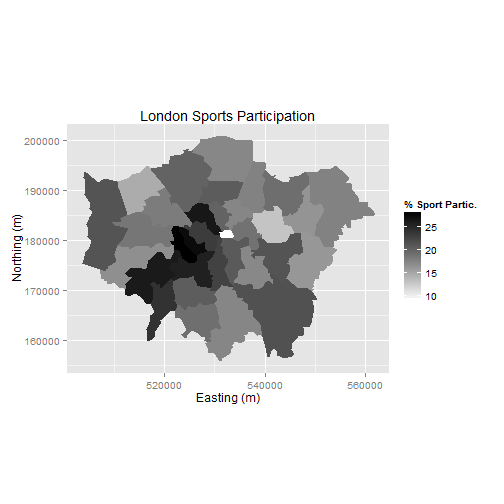Saving plot images is also easy. You just need to use ggsave after each plot, e.g. `ggsave("my_map.pdf")` will save the map as a pdf, with default settings. For a larger map, you could try the following:

``````ggsave("my_large_plot.png", scale = 3, dpi = 400)
``````
``````## Saving 21 x 21 in image
``````

### Adding base maps to ggplot2 with ggmap

`ggmap` is a package that uses the ggplot2 syntax as a template to create maps with image tiles from the likes of Google and OpenStreetMap:

``````library(ggmap)  # you may have to use install.packages to install it first
``````

The sport object is in British National Grid but the ggmap image tiles are in `WGS84`. We therefore need to use the `sport.wgs84` object created in the reprojection operation earlier.

The first job is to calculate the bounding box (`bb` for short) of the `sport.wgs84` object to identify the geographic extent of the image tiles that we need.

``````b <- bbox(sport.wgs84)
b[1, ] <- (b[1, ] - mean(b[1, ])) * 1.05 + mean(b[1, ])
b[2, ] <- (b[2, ] - mean(b[2, ])) * 1.05 + mean(b[2, ])
# scale longitude and latitude (increase bb by 5% for plot) replace 1.05
# with 1.xx for an xx% increase in the plot size
``````

This is then fed into the get_map function as the location parameter. The syntax below contains 2 functions. `ggmap` is required to produce the plot and provides the base map data.

``````lnd.b1 <- ggmap(get_map(location = b))
``````
``````## Warning: bounding box given to google - spatial extent only approximate.
``````
``````## converting bounding box to center/zoom specification. (experimental)
## Map from URL : http://maps.googleapis.com/maps/api/staticmap?center=51.489304,-0.088233&zoom=10&size=%20640x640&scale=%202&maptype=terrain&sensor=false
``````

In much the same way as we did above we can then layer the plot with different geoms.

First fortify the `sport.wgs84` object and then merge with the required attribute data (we already did this step to create the `sport.f` object).

``````sport.wgs84.f <- fortify(sport.wgs84, region = "ons_label")
sport.wgs84.f <- merge(sport.wgs84.f, sport.wgs84@data, by.x = "id", by.y = "ons_label")
``````

The code above contains a lot of parameters. Use the ggplot2 help pages to find out what they are. The resulting map looks okay, but it would be improved with a simpler base map in black and white. A design firm called stamen provide the tiles we need and they can be brought into the plot with the `get_map` function:

``````lnd.b2 <- ggmap(get_map(location = b, source = "stamen", maptype = "toner",
crop = T))
``````

We can then produce the plot as before.

``````lnd.b2 + geom_polygon(data = sport.wgs84.f, aes(x = long, y = lat, group = group,
fill = Partic_Per), alpha = 0.5)
``````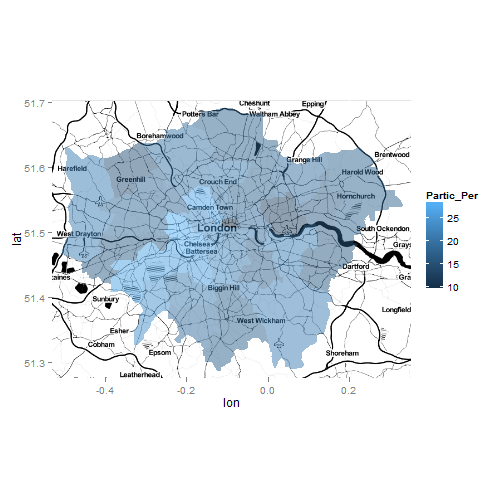Finally, if we want to increase the detail of the base map, `get_map` has a zoom parameter.

``````lnd.b3 <- ggmap(get_map(location = b, source = "stamen", maptype = "toner",
crop = T, zoom = 11))  # 3 = Continent, 10 = City (default), 21 = Building

lnd.b3 + geom_polygon(data = sport.wgs84.f, aes(x = long, y = lat, group = group,
fill = Partic_Per), alpha = 0.5)
``````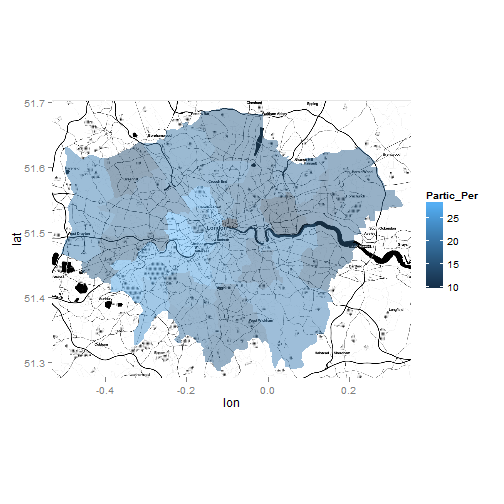### Joining and clipping

This section builds on the previous information on plotting and highlights some of R's more advanced spatial functions from the `rgeos` package. We look at joining new datasets to our data - an attribute join - spatial joins, whereby data is added to the target layer depending on the location of the origins and clipping.

To reaffirm our starting point, let's re-plot the only spatial dataset in our workspace, and count the number of polygons:

``````library(rgdal)
lnd <- readOGR(dsn = ".", "london_sport")
``````
``````## OGR data source with driver: ESRI Shapefile
## Source: ".", layer: "london_sport"
## with 33 features and 4 fields
## Feature type: wkbPolygon with 2 dimensions
``````
``````plot(lnd)
``````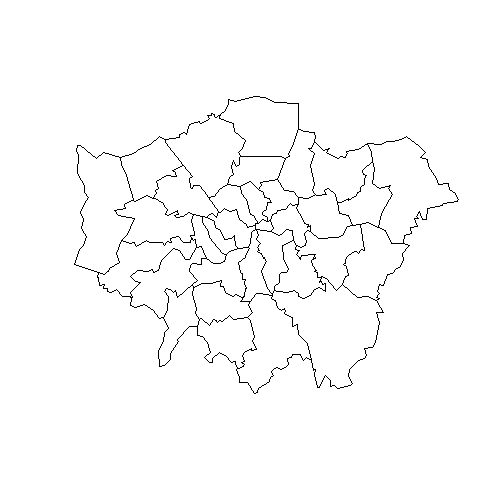``````nrow(lnd)
``````
``````##  33
``````

Because we are using borough-level data, and boroughs are official administrative zones, there is much data available at this level. We will use the example of crime data to illustrate this data availability, and join this with the current spatial dataset. As before, we can download and import the data from within R:

``````download.file("http://data.london.gov.uk/datafiles/crime-community-safety/mps-recordedcrime-borough.csv",
destfile = "mps-recordedcrime-borough.csv")

crimeDat <- read.csv("mps-recordedcrime-borough.csv")  # flags an error
``````

Initially, the `read.csv` command flags an error: open the raw .csv file in a text editor such as Notepad, Notepad++ or GVIM, find the problem and correct it. Alternatively, you can work out what the file encoding is and use the correct argument (this is not recommended - simpler just to edit the text file in most cases).

``````crimeDat <- read.csv("mps-recordedcrime-borough.csv", fileEncoding = "UCS-2LE")
``````
``````##    Month                   MajorText                   MinorText
## 1 201104 Violence Against The Person              Common Assault
## 2 201104                    Burglary      Burglary In A Dwelling
## 3 201104   Other Notifiable Offences            Other Notifiable
## 4 201104                     Robbery           Personal Property
## 5 201104            Theft & Handling       Handling Stolen Goods
## 6 201104            Theft & Handling Theft/Taking Of Pedal Cycle
##   CrimeCount   Spatial_DistrictName
## 1         81 Kensington and Chelsea
## 2         78 Kensington and Chelsea
## 3         12 Kensington and Chelsea
## 4         41 Kensington and Chelsea
## 5          3 Kensington and Chelsea
## 6         59 Kensington and Chelsea
``````
``````summary(crimeDat\$MajorText)
``````
``````##                    Burglary             Criminal Damage
##                        1543                        3125
##                       Drugs             Fraud & Forgery
##                        1925                        1581
##   Other Notifiable Offences                     Robbery
##                        1374                        1517
##             Sexual Offences            Theft & Handling
##                        1557                        6264
## Violence Against The Person
##                        4885
``````
``````crimeTheft <- crimeDat[which(crimeDat\$MajorText == "Theft & Handling"), ]
head(crimeTheft, 2)  # change 2 for more rows
``````
``````##    Month        MajorText                   MinorText CrimeCount
## 5 201104 Theft & Handling       Handling Stolen Goods          3
## 6 201104 Theft & Handling Theft/Taking Of Pedal Cycle         59
##     Spatial_DistrictName
## 5 Kensington and Chelsea
## 6 Kensington and Chelsea
``````
``````crimeAg <- aggregate(CrimeCount ~ Spatial_DistrictName, FUN = "sum", data = crimeTheft)
head(crimeAg, 2)  # show the aggregated crime data
``````
``````##   Spatial_DistrictName CrimeCount
## 1 Barking and Dagenham      12222
## 2               Barnet      19821
``````

Now that we have crime data at the borough level, the challenge is to join it by name. This is not always straightforward. Let us see which names in the crime data match the spatial data:

``````lnd\$name %in% crimeAg\$Spatial_DistrictName
``````
``````##    TRUE  TRUE  TRUE  TRUE  TRUE  TRUE  TRUE  TRUE  TRUE  TRUE  TRUE
##   TRUE  TRUE  TRUE  TRUE  TRUE  TRUE  TRUE  TRUE  TRUE  TRUE  TRUE
##   TRUE  TRUE  TRUE  TRUE  TRUE  TRUE  TRUE  TRUE  TRUE  TRUE FALSE
``````
``````lnd\$name[which(!lnd\$name %in% crimeAg\$Spatial_DistrictName)]
``````
``````##  City of London
## 33 Levels: Barking and Dagenham Barnet Bexley Brent Bromley ... Westminster
``````

The first line of code above shows that all but one of the borough names matches; the second tells us that it is City of London that is named differently in the crime data. Look at the results (not shown here) on your computer.

``````levels(crimeAg\$Spatial_DistrictName)
``````
``````##   "Barking and Dagenham"   "Barnet"
##   "Bexley"                 "Brent"
##   "Bromley"                "Camden"
##   "Croydon"                "Ealing"
##   "Enfield"                "Greenwich"
##  "Hackney"                "Hammersmith and Fulham"
##  "Haringey"               "Harrow"
##  "Havering"               "Hillingdon"
##  "Hounslow"               "Islington"
##  "Kensington and Chelsea" "Kingston upon Thames"
##  "Lambeth"                "Lewisham"
##  "Merton"                 "Newham"
##  "NULL"                   "Redbridge"
##  "Richmond upon Thames"   "Southwark"
##  "Sutton"                 "Tower Hamlets"
##  "Waltham Forest"         "Wandsworth"
##  "Westminster"
``````
``````levels(crimeAg\$Spatial_DistrictName) <- as.character(lnd\$name[which(!lnd\$name %in%
crimeAg\$Spatial_DistrictName)])
lnd\$name %in% crimeAg\$Spatial_DistrictName  # now all columns match
``````
``````##   TRUE TRUE TRUE TRUE TRUE TRUE TRUE TRUE TRUE TRUE TRUE TRUE TRUE TRUE
##  TRUE TRUE TRUE TRUE TRUE TRUE TRUE TRUE TRUE TRUE TRUE TRUE TRUE TRUE
##  TRUE TRUE TRUE TRUE TRUE
``````

The above code block first identified the row with the faulty name and then renamed the level to match the lnd dataset. Note that we could not rename the variable directly, as it is stored as a factor.

We are now ready to join the datasets. It is recommended to use the join function in the plyr package but the merge function could equally be used.

``````library(plyr)
``````

The documentation for join will be displayed if the plyr package is loaded (if not, load or install and load it!). It requires all joining variables to have the same name, so we will rename the variable to make the join work:

``````head(lnd\$name)
``````
``````##  Bromley              Richmond upon Thames Hillingdon
##  Havering             Kingston upon Thames Sutton
## 33 Levels: Barking and Dagenham Barnet Bexley Brent Bromley ... Westminster
``````
``````head(crimeAg\$Spatial_DistrictName)  # the variables to join
``````
``````##  Barking and Dagenham Barnet               Bexley
##  Brent                Bromley              Camden
## 33 Levels: Barking and Dagenham Barnet Bexley Brent Bromley ... Westminster
``````
``````crimeAg <- rename(crimeAg, replace = c(Spatial_DistrictName = "name"))
head(join(lnd@data, crimeAg))  # test it works
``````
``````## Joining by: name
``````
``````##   ons_label                 name Partic_Per Pop_2001 CrimeCount
## 1      00AF              Bromley       21.7   295535      15172
## 2      00BD Richmond upon Thames       26.6   172330       9715
## 3      00AS           Hillingdon       21.5   243006      15302
## 4      00AR             Havering       17.9   224262      12611
## 5      00AX Kingston upon Thames       24.4   147271       9023
## 6      00BF               Sutton       19.3   179767       8810
``````
``````
lnd@data <- join(lnd@data, crimeAg)
``````
``````## Joining by: name
``````

### Adding point data for clipping and spatial join

In addition to joining by zone name, it is also possible to do spatial joins in R. There are three main varieties: many-to-one - where the values of many intersecting objects contribute to a new variable in the main table - one-to-many, or one-to-one. Because boroughs in London are quite large, we will conduct a many-to-one spatial join. We will be using Tube Stations as the spatial data to join, with the aim of finding out which and how many stations are found in each London borough.

``````download.file("http://www.personal.leeds.ac.uk/~georl/egs/lnd-stns.zip", "lnd-stns.zip")
unzip("lnd-stns.zip")
library(rgdal)
stations <- readOGR(dsn = ".", layer = "lnd-stns", p4s = "+init=epsg:27700")
``````
``````## OGR data source with driver: ESRI Shapefile
## Source: ".", layer: "lnd-stns"
## with 2532 features and 27 fields
## Feature type: wkbPoint with 2 dimensions
``````
``````proj4string(stations)  # this is the full geographical detail.
``````
``````##  "+init=epsg:27700 +proj=tmerc +lat_0=49 +lon_0=-2 +k=0.9996012717 +x_0=400000 +y_0=-100000 +datum=OSGB36 +units=m +no_defs +ellps=airy +towgs84=446.448,-125.157,542.060,0.1502,0.2470,0.8421,-20.4894"
``````
``````proj4string(lnd)
``````
``````##  "+proj=tmerc +lat_0=49 +lon_0=-2 +k=0.9996012717 +x_0=400000 +y_0=-100000 +ellps=airy +units=m +no_defs"
``````
``````bbox(stations)
``````
``````##              min    max
## coords.x1 455435 602523
## coords.x2 122266 227704
``````
``````bbox(lnd)
``````
``````##      min    max
## x 503571 561941
## y 155851 200932
``````

The above code loads the data correctly, but also shows that there are problems with it: the Coordinate Reference System (CRS) differs from that of our shapefile. Although OSGB 1936 (or EPSG 27700) is the 'correct' CRS for the UK, we will convert the stations dataset into lat-long coordinates, as this is a more common CRS and enables easy base map creation:

``````stationsWGS <- spTransform(stations, CRSobj = CRS(proj4string(lnd)))
stations <- stationsWGS
rm(stationsWGS)
plot(lnd)
points(stations[sample(1:nrow(stations), size = 500), ])
``````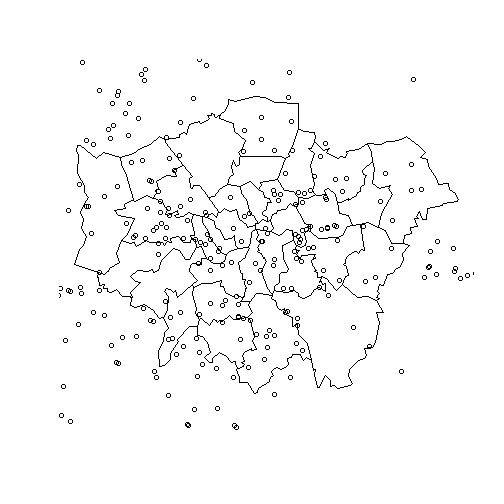Now we can clearly see that the stations overlay the boroughs. The problem is that the stations dataset is far more extensive than London borough dataset; we need

### Clipping

There are a number of functions that we can use to clip the points so that only those falling within London boroughs are retained:

```````?`(overlay)
`?`(sp::over)
library(rgeos)
`?`(rgeos::gIntersects)
``````

We can write off the first one straight away as it is depreciated by the second. It seems that `gIntersects` can produce the same output as over, based on discussion in the community, so either can be used. (See this discussion for further alternatives.) In this tutorial we will use gIntersects, for clipping although we could equally use gContains, `gWithin` and other g… functions - see rgeos help pages by typing `?gOverlaps` or other functions for more. `gIntersects` will output information for each point, telling us which polygon it interacts with (i.e. the polygon it is in):

``````int <- gIntersects(stations, lnd, byid = T)  # find which stations intersect
class(int)  # it's outputed a matrix
``````
``````##  "matrix"
``````
``````dim(int)  # with 33 rows (one for each zone) and 2532 cols (the points)
``````
``````##    33 2532
``````
``````summary(int[, c(200, 500)])  # not the output of this
``````
``````##     200             500
##  Mode :logical   Mode :logical
##  FALSE:33        FALSE:32
##  NA's :0         TRUE :1
##                  NA's :0
``````
``````plot(lnd)
points(stations[200, ], col = "red")  # note point id 200 is outside the zones
points(stations[500, ], col = "green")  # note point 500 is inside
which(int[, 500] == T)  # this tells us that point 500 intersects with zone 32
``````
``````## 31
## 32
``````
``````points(coordinates(lnd[32, ]), col = "black")  # test the previous statement
``````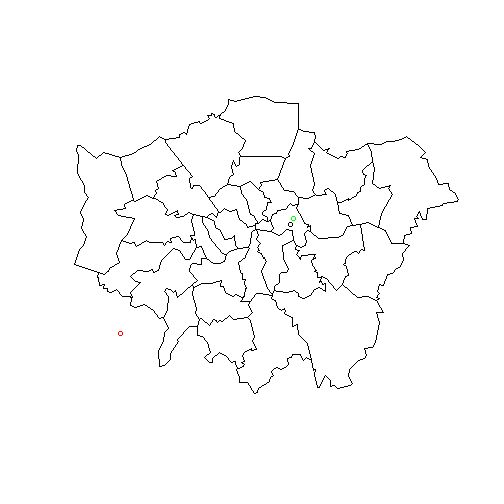In the above code, only the first line actually 'does' anything in our workspace, by creating the object `int`. The proceeding lines are dedicated to exploring this object and what it means. Note that it is a matrix with columns corresponding to the points and rows corresponding to boroughs. The borough in which a particular point can be extracted from int as we shall see below. For the purposes of clipping, we are only interested in whether the point intersects with any of the boroughs. This is where the function `apply`, which is unique to R, comes into play:

``````clipped <- apply(int == F, MARGIN = 2, all)
plot(stations[which(clipped), ])  # shows all stations we DO NOT want
stations.cl <- stations[which(!clipped), ]  # use ! to select the invers
points(stations.cl, col = "green")  # check that it's worked
``````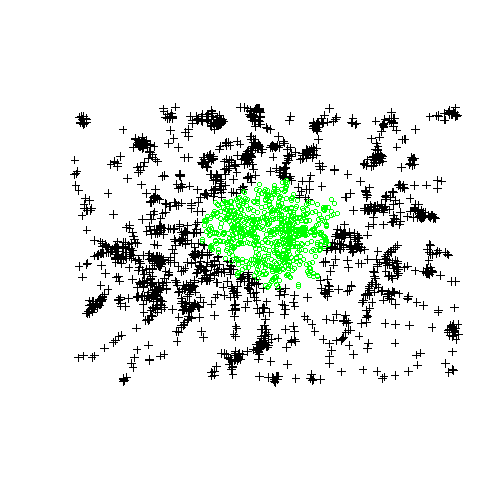``````stations <- stations.cl
rm(stations.cl)  # tidy up: we're only interested in clipped ones
``````

The first line instructs R to look at each column (`MARGIN = 2`, we would use `MARGIN = 1` for row-by-row analysis) and report back whether all of the values are false. This creates the inverse selection that we want, hence the use of `!` to invert it. We test that the function works on a new object (often a good idea, to avoid overwriting useful data) with plots and, once content that the clip has worked, save the sample of points to our main stations object and remove the now duplicated stations.cl object.

### Aggregating the data to complete the spatial join

Now that we know how `gIntersects` works in general terms and for clipping, let's use it to allocate a borough to each of our station points, which we will then aggregate up. Data from these points (e.g. counts, averages in each area etc.) can then be transferred to the main polygons table: the essence of a spatial join. Again, `apply` is our friend in this instance, meaning we can avoid for loops:

``````int <- gIntersects(stations, lnd, byid = T)  # re-run the intersection query
head(apply(int, MARGIN = 2, FUN = which))
``````
``````## 91 92 93 94 95 96
##  6 10 10 10 10 10
``````
``````b.indexes <- which(int, arr.ind = T)
summary(b.indexes)
``````
``````##       row          col
##  Min.   : 1   Min.   :  1
##  1st Qu.: 7   1st Qu.:183
##  Median :15   Median :366
##  Mean   :15   Mean   :366
##  3rd Qu.:22   3rd Qu.:548
##  Max.   :33   Max.   :730
``````
``````b.names <- lnd\$name[b.indexes[, 1]]
b.count <- aggregate(b.indexes ~ b.names, FUN = length)
``````
``````##                b.names row col
## 1 Barking and Dagenham  12  12
## 2               Barnet  29  29
## 3               Bexley  28  28
## 4                Brent  30  30
## 5              Bromley  54  54
## 6               Camden  14  14
``````

The above code first extracts the index of the row (borough) for which the corresponding column is true and then converts this into names. The final object created, `b.count` contains the number of station points in each zone. According to this, Barking and Dagenham should contain 12 station points. It is important to check the output makes sense at every stage with R, so let's check to see this is indeed the case with a quick plot:

``````plot(lnd[which(grepl("Barking", lnd\$name)), ])
points(stations)
``````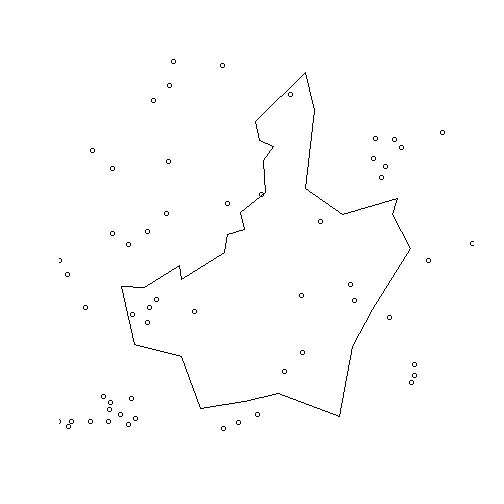Now the fun part: count the points in the polygon and report back how many there are!

The final stage is to transfer the data on station counts back into the polygon data frame. We have used merge to join two datasets before. In R there is often more than one way to achieve the same result. It's good to experiment with different functions, so we will use `join` from the plyr package. join requires identical joining names in both data frames, so first we will rename them (type `?rename` for more details).

``````b.count <- rename(b.count, replace = c(b.names = "name"))
b.count.tmp <- join(lnd@data, b.count)
``````
``````## Joining by: name
``````
``````
``````
``````##   ons_label                 name Partic_Per Pop_2001 CrimeCount row col
## 1      00AF              Bromley       21.7   295535      15172  54  54
## 2      00BD Richmond upon Thames       26.6   172330       9715  22  22
``````
``````
lnd\$station.count <- b.count.tmp[, 7]
``````

We have now seen how to join and clip data. Next, for a stronger grounding in how ggplot works, we will look at plotting non-spatial data.

### Using ggplot2 for Descriptive Statistics

For this we will use a new dataset:

``````input <- read.csv("ambulance_assault.csv")
``````

This contains the number of ambulance callouts to assault incidents (downloadable from the London DataStore) between 2009 and 2011.

Take a look at the contents of the file:

``````head(input)
``````
``````##   Bor_Code     WardName WardCode assault_09_11
## 1     00AA   Aldersgate   00AAFA            10
## 2     00AA      Aldgate   00AAFB             0
## 3     00AA    Bassishaw   00AAFC             0
## 4     00AA Billingsgate   00AAFD             0
## 5     00AA  Bishopsgate   00AAFE           188
## 6     00AA Bread Street   00AAFF             0
``````

We can now plot a histogram to show the distribution of values.

``````p.ass <- ggplot(input, aes(x = assault_09_11))
``````

The resulting message (stat_bin: binwidth defaulted to range/30…) relates to the bins - the breaks between histogram blocks. If you want the bins (and therefore the bars) to be thinner (i.e. representing fewer values) you need to make the bins smaller by adjusting the binwidth. Try:

``````p.ass + geom_histogram(binwidth = 10) + geom_density(fill = NA, colour = "black")
``````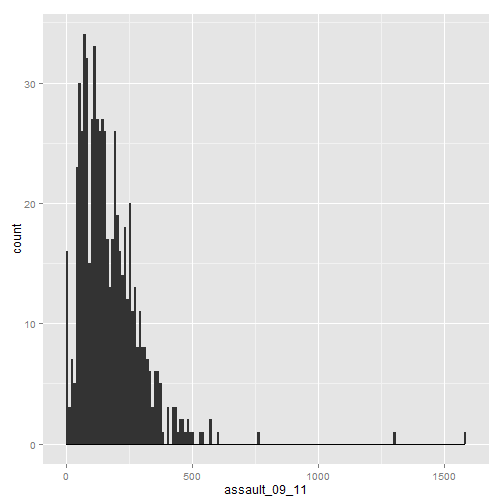It is also possible to overlay a density distribution over the top of the histogram. For this we need to produce a second plot object with the density distribution as the y variable.

``````p2.ass <- ggplot(input, aes(x = assault_09_11, y = ..density..))

p2.ass + geom_histogram() + geom_density(fill = NA, colour = "red")
``````
``````## stat_bin: binwidth defaulted to range/30. Use 'binwidth = x' to adjust this.
``````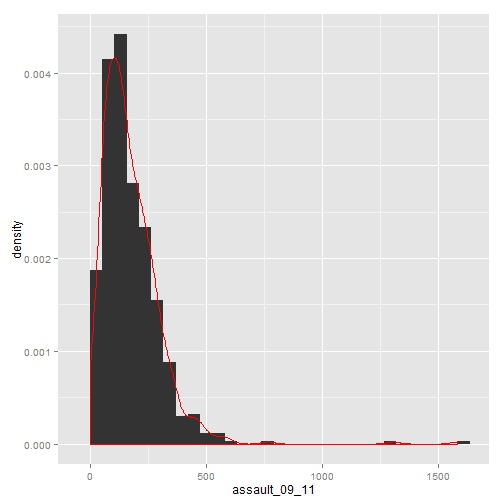What kind of distribution is this plot showing? You can see that there are a few wards with very high assault incidences (over 750). To find out which ones these are we can select them.

``````input[which(input\$assault_09_11 > 750), ]
``````
``````##     Bor_Code   WardName WardCode assault_09_11
## 153     00AH  Fairfield   00AHGM           765
## 644     00BK St James's   00BKGQ          1582
## 649     00BK   West End   00BKGW          1305
``````

It is perhaps unsurprising that St James's and the West End have the highest counts. The plot has provided a good impression of the overall distribution, but what are the characteristics of each distribution within the Boroughs? Another type of plot that shows the core characteristics of the distribution is a box and whisker plot. These too can be easily produced in R (you can't do them in Excel!). We can create a third plot object (note that the assault field is now y and not x):

``````p3.ass <- ggplot(input, aes(x = Bor_Code, y = assault_09_11))
``````

and convert it to a boxplot.

``````p3.ass + geom_boxplot()
``````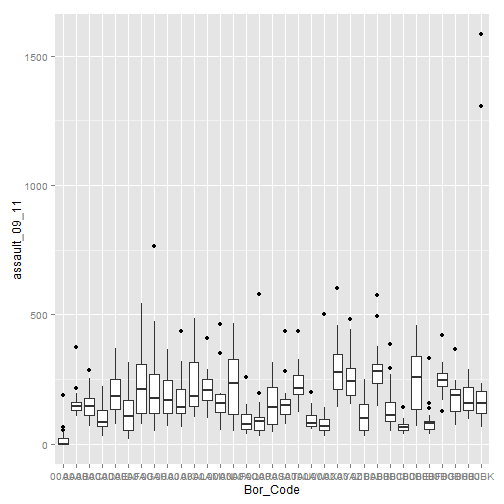Perhaps this would look a little better flipped round.

``````p3.ass + geom_boxplot() + coord_flip()
``````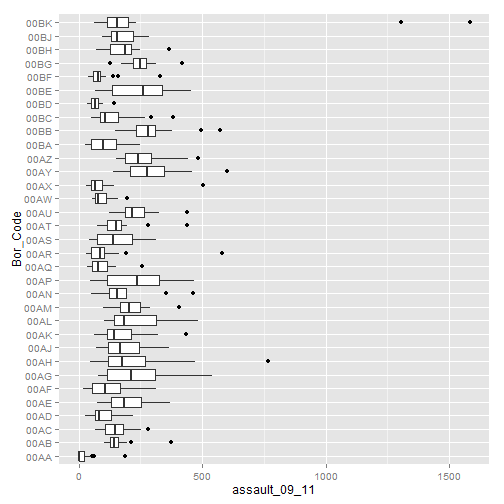Now each of the borough codes can be easily seen. No surprise that the Borough of Westminster (00BK) has the two largest outliers. In one line of code you have produced an incredibly complex plot rich in information. This demonstrates why R is such a useful program for these kinds of statistics.

If you want an insight into some of the visualisations you can develop with this type of data we can do faceting based on the example of the histogram plot above.

``````p.ass + geom_histogram() + facet_wrap(~Bor_Code)
``````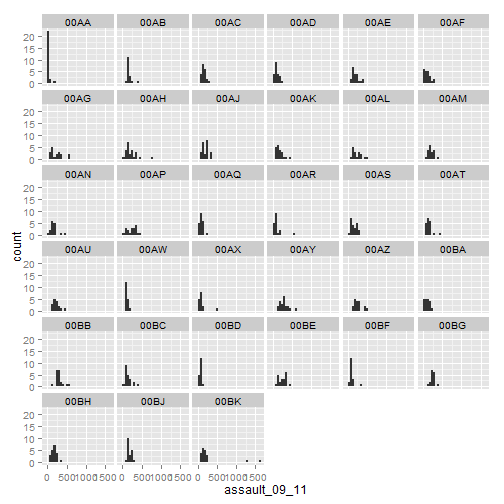We need to do a little bit of tweaking to make this plot publishable but we want to demonstrate that it is really easy to produce 30+ plots on a single page! Faceting is an extremely powerful way of visualizing multidimensional datasets and is especially good for showing change over time.

``````library(reshape2)  # this may not be installed.
# If not install it, or skip the next two steps…
``````

Load the data - this shows historic population values between 1801 and 2001 for London, again from the London data store.

``````london.data <- read.csv("census-historic-population-borough.csv")
``````

“Melt” the data so that the columns become rows.

``````london.data.melt <- melt(london.data, id = c("Area.Code", "Area.Name"))
``````

Only do this step if reshape and melt failed

``````# london.data.melt <- read.csv('london_data_melt.csv')
``````

Merge the population data with the London borough geometry contained within our `sport.f` object.

``````plot.data <- merge(sport.f, london.data.melt, by.x = "id", by.y = "Area.Code")
``````

Reorder this data (ordering is important for plots).

``````plot.data <- plot.data[order(plot.data\$order), ]
``````

We can now use faceting to produce one map per year (this may take a little while to appear).

``````ggplot(data = plot.data, aes(x = long, y = lat, fill = value, group = group)) +
geom_polygon() + geom_path(colour = "grey", lwd = 0.1) + coord_equal() +
facet_wrap(~variable)
``````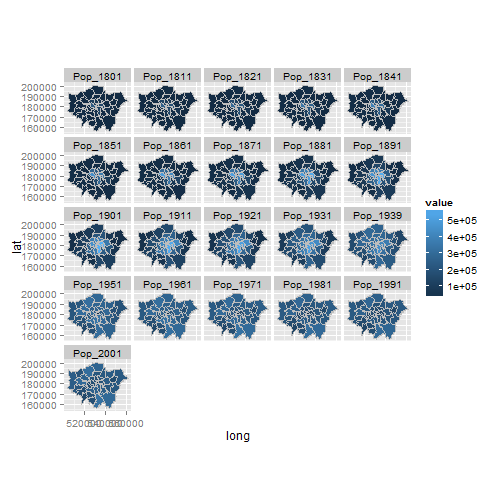Again there is a lot going on here so explore the documentation to make sure you understand it. Try out different colour values as well.

Add a title and replace the axes names with “easting” and “northing” and save your map as a pdf.

### Taking spatial data analysis in R further

The skills you have learned in this tutorial are applicable to a very wide range of datasets, spatial or not. Often experimentation is the most rewarding learning method, rather than just searching for the 'best' way of doing something (Kabakoff, 2011). We recommend you play around with your own data.

The supportive online communities surrounding large open source programs such as R are one of their greatest assets, so we recommend you become an active “open source citizen” rather than a passive consumer (Ramsey & Dubovsky, 2013).

This does not necessarily mean writing R source code - it can simply mean helping others use R. We therefore conclude the tutorial with a list of resources that will help you further sharpen you R skills, find help and contribute to the growing online R community:

• R's homepage hosts a wealth of official and contributed guides.
• Stack Exchange and GIS Stack Exchange groups - try searching for “[R]”. If your issue has not been not been addressed yet, you could post a polite question.
• R's mailing lists - the R-sig-geo list may be of particular interest here.

Books: despite the strength of R's online community, nothing beats a physical book for concentrated learning. We would particularly recommend the following:

• ggplot2: elegant graphics for data analysis (Wickham 2009)
• Bivand et al. (2013) Provide a dense and detailed overview of spatial data analysis in an updated version of the book by the developers of many of R's spatial functions.
• Kabacoff (2011) is a more general R book; it has many fun worked examples.

### References

Bivand, R. S., Pebesma, E. J., & Rubio, V. G. (2008). Applied spatial data: analysis with R. Springer.

Harris, R. (2012). A Short Introduction to R. social-statistics.org.

Kabacoff, R. (2011). R in Action. Manning Publications Co.

Ramsey, P., & Dubovsky, D. (2013). Geospatial Software's Open Future. GeoInformatics, 16(4).

Torfs and Brauer (2012). A (very) short Introduction to R. The Comprehensive R Archive Network.

Wickham, H. (2009). ggplot2: elegant graphics for data analysis. Springer.

Wilkinson, L. (2005). The grammar of graphics. Springer.

``````sessionInfo()
``````
``````## R version 3.0.2 (2013-09-25)
## Platform: x86_64-w64-mingw32/x64 (64-bit)
##
## locale:
##  LC_COLLATE=English_United Kingdom.1252
##  LC_CTYPE=English_United Kingdom.1252
##  LC_MONETARY=English_United Kingdom.1252
##  LC_NUMERIC=C
##  LC_TIME=English_United Kingdom.1252
##
## attached base packages:
##  stats     graphics  grDevices utils     datasets  methods   base
##
## other attached packages:
##   reshape2_1.2.2  plyr_1.8        mapproj_1.2-1   maps_2.3-6
##   ggmap_2.3       rgeos_0.3-2     maptools_0.8-27 ggplot2_0.9.3.1
##   rgdal_0.8-14    sp_1.0-14       knitr_1.5
##
## loaded via a namespace (and not attached):
##   colorspace_1.2-4    dichromat_2.0-0     digest_0.6.4
##   evaluate_0.5.1      foreign_0.8-55      formatR_0.10
##   grid_3.0.2          gtable_0.1.2        labeling_0.2
##  lattice_0.20-24     MASS_7.3-29         munsell_0.4.2
##  png_0.1-7           proto_0.3-10        RColorBrewer_1.0-5